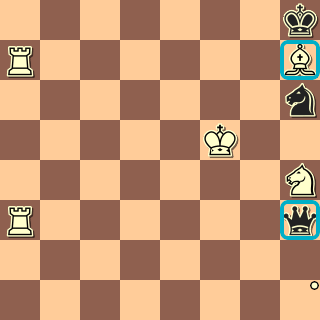# Square variables

A square variable is a variable whose value is a square on the chessboard. The name of the variable must begin with '\$'. A square variable is introduced using the `square` filter```square \$x in h1-8
attack (R \$x)
attack (\$x K)```
This filter is associated with the set of squares on the h file that are both attacked by a white rook and contain a piece that attacks the square occupied by the white King. Note that:

`qh3` attacks the white king's square and is attacked by a white rook - match!
`nh6` attacks the white king's square but is NOT attacked by the white rook - no match
`Nh4` attacks the white king's square but is NOT attacked by the white rook - no match
`Bh7` attacks the white king's square and is attacked by Ra7 - match!

all other squares on the h-file fail both clauses The result is that filter matches when `\$x` is either `h3` or `h7`. `\$x` is used to refer to a square within the body of the square filter. The square variable is bound to each square on the h file successively, and each of the forms in the body of the square filter are evaluated for that binding. If each form is true, then the square bound to \$x is a member of the set associated with the filter.

## syntax of the square filter

The square filter has the following basic syntax:
```square variable_name in in_set
filter_1
filter_2
...```
As usual, by "filter_1 filter_2..." we just mean one or more filters - the second filter can be missing.

The filter is associated with the a set S defined as follows. A square s is in S (in the current position) if, when the variable variable_name is replaced by the square s in each of the forms filter_1, filter_2..., then each of those filters are true.

In the example, above, for example, the square h5 is in the set associated with the square filter only if

`attack(R h5)`
is true, and also
`attack (h5 K)`
is true. Note that the first clause could be true if h5 was an empty square, but the second clause can only be true if h5 has any piece of any color except a king.

## using ranges with square filters

There are three ways to use a range with a square filter to count the number of elements in the set the filter represents.

### Using countsquares with square filters

First, since a square filter returns a set like any other, it can be counted using `countsquares`.

```countsquares 0
square \$x in A
attack (\$x k)```
means that the black King is not in check, because the number of squares on which there is a white piece that attacks the black king equals 0.

Similarly,

```countsquares 2 3
square \$x in h1-8
R on \$x```
is a somewhat long-winded way of writing that there between 2 and 3 rooks on the h file. (This can be more simply written as `countsquares 2 3 Rh1-8`).

### Using a range after the word 'square'

Second, if the word 'square' is immediately followed by a range, then the filter is true only if the number of elements in the set the filter represents lies within the range.

For example,

`square 2 5 \$x in h1-8 attack (R \$x) attack (\$x K)`
matches the current position only if there are between 2 and 5 squares on the h file that are both attacked by an R and attack the K. This is equivalent to:
```countsquares 2 5
square \$x in h1-8
attack (R \$x)
attack (\$x K)```

### Using the keyword 'all' after the word 'square'

Third, the keyword 'all' is a special range that is equivalent to the number of elements in the set following the word `in`. For example,
```square all \$x in attack (k _)
attack (A \$x)```
means that each empty square in the black king's field is attacked by a white piece. To find pure mates, where each such square is attacked only once, we would use:
```square all \$x in attack (k _)
attack 1 (A \$x)```

Examples